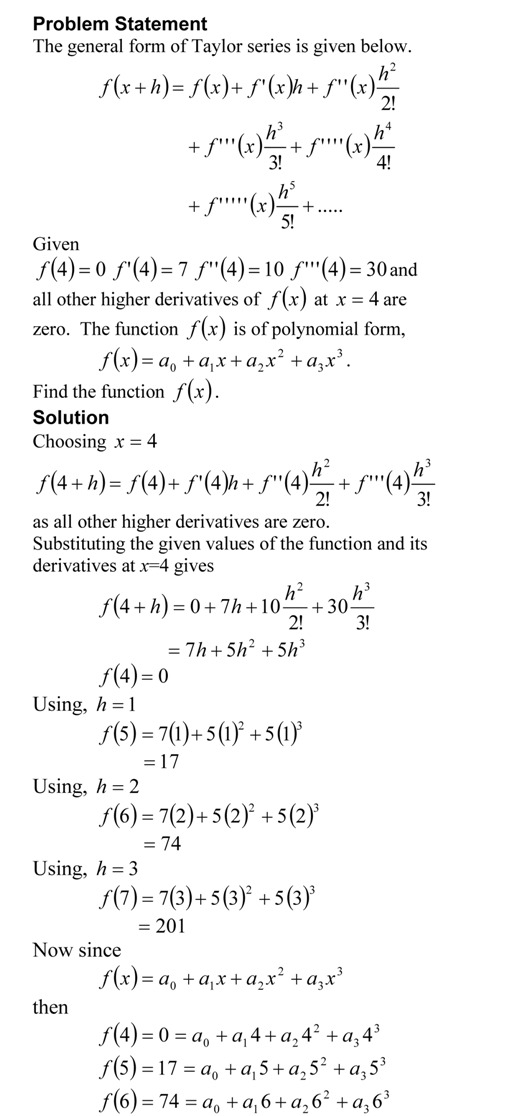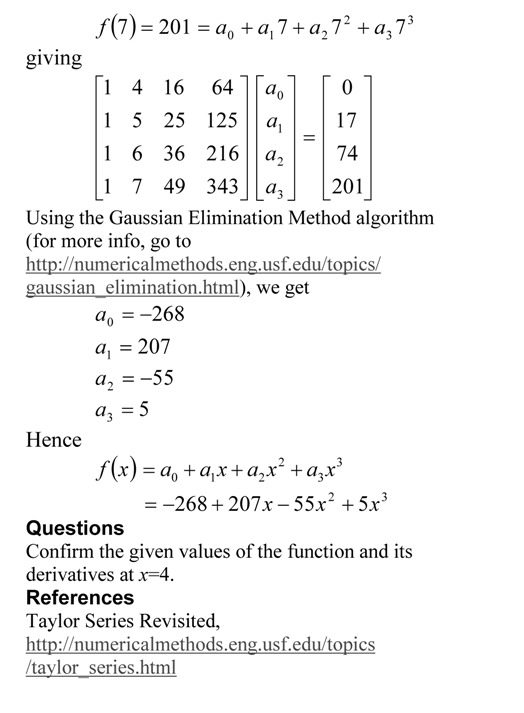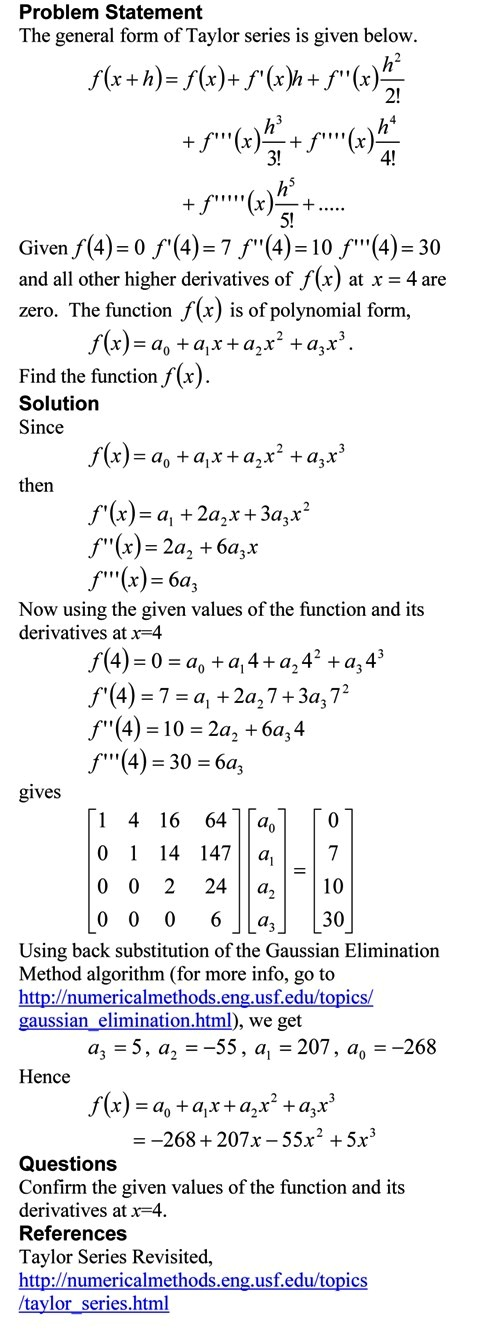## Computational Time for Back Substitution

This is a blog that will show you how we can find the approximate time it takes to conduct back substitution, while solving simultaneous linear equations using Gaussian elimination method. The blog assumes a AMD-K7 2.0GHz chip that uses 4 clock cycles for addition, subtraction and multiplication, and 16 clock cycles for division. Note that we are making reasonable approximations in this blog. Our main motto is to find how the computational time is related to the number of equations.The pdf file of the solution is also available.

This post is brought to you by

## Taylor Series Exercise – Method 3

Taylor series is an important concept for learning numerical methods – not only for understanding how trigonometric and transcendental functions are calculated by a computer, but also for error analysis in numerical methods. I asked the question below in the first test in the course, and half of the students did not get to the final answer. In a previous blog, I showed you the method that most instructors would use. See how some students approached (another approach) the problem.The pdf file of the solution is also available.

This post is brought to you by